# Seed germination

Seed germination is 80%. 8% of the germinated plants subsequently die. How many seeds does a gardener have to sow in order to grow 2,000 plants?

n =  2718

### Step-by-step explanation: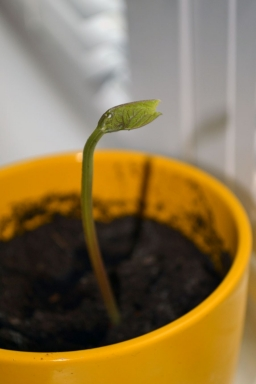Did you find an error or inaccuracy? Feel free to write us. Thank you!Tips to related online calculators
Need help to calculate sum, simplify or multiply fractions? Try our fraction calculator.
Do you have a linear equation or system of equations and looking for its solution? Or do you have a quadratic equation?
Do you want to round the number?

## Related math problems and questions:

• Carrot seed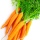Carrot seed germination is 85%, weight 1000 carrots seeds are 2.4g. How many seeds are likely to germinate if we sow 8g of seeds?
• Alcohol mixtureFrom 55% and 80% alcohol, we have to produce 0.2 kg of 60% alcohol. How many of them do we use in the solution?
• Alcohol solutionsWe have to produce 2 liters of 60% alcohol from 55% and 80%. How many of which ones will we use in the solution?
• SeedsFrom a box of spruce seeds with the germination of 80%, we randomly select 10 seeds and plant them. Find the median of the random variable: the number of germinating seeds.
• SolutionWhat amount of 80% solution and what amount of water is needed to mix in order to obtain two liters of 60% solution of this mixture?
• Acid evaporationHow many kilograms of water do we have to evaporate from 100 kg of 32% acid to make it 80% concentration?
• Apples 2Dried apples contain 15% water. Fresh apples contain 80% water. How many kg of apples we need buy in order to get 3 kg of dried apples?
• SpiritFrom 55% and 80% spirit we would like to produce 0.2 kg of 60% spirit. How many of them we must use in a solution?
• Cinema ticketsCinema sold 180 tickets this Thursday, which is 20%. Monday 14%, Tuesday 6%, Wednesday 9%, Friday 24%, Saturday 12%, and Sunday 15%. How many tickets were sold per week?
• Seeds 2How many seeds germinated from 1000 pcs, when 23% no emergence?
• Gp - 80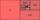One of the first four members of a geometric progression is 80. Find its if we know that the fourth member is nine times greater than the second.
• Test pointsIf you earned 80% of the possible 40 points, how many points did you miss to get 100%?
• The city 2Today lives 298000 citizens in the city. How many citizens can we expect in 8 years if their annual increase is 2.4%?
• Percent chaining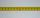Calculate 20% from 70% from 80 km
• Salary in enterprise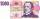The average salary in the company is 27 000 CZK, 30% of workers have the lowest average income of 19 thousand CZK. There were an increase in the salary in this group by 2%. How much % increased the average salary across the company?
• Alcohol mixing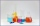How much 64% of the alcohol must be poured into 6 liters of 85% alcohol to produce 79% alcohol?
• CrystalThe crystal grows every month 1.2 permille of its mass. For how many months to grow a crystal from weight 177 g to 384 g?